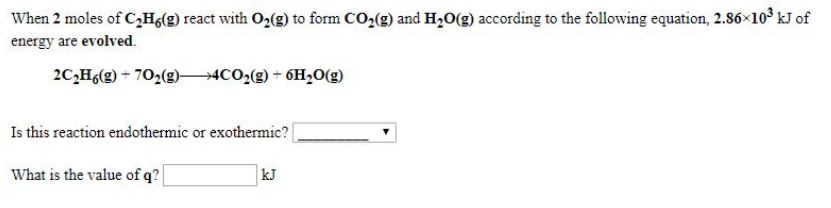# Problem: When 2 moles of C2H6 (g) react with O2 (g) to form CO2 (g) and H2O (g) according to the following equation, 2.86 x 103 kJ of energy are evolved. 2C2H6 (g) + 7O2 (g) → 4CO2 (g) + 6H2O(g) Is this reaction endothermic or exothermic? What is the value of q?

###### FREE Expert Solution
80% (107 ratings)###### Problem Details

When 2 moles of C2H6 (g) react with O2 (g) to form CO2 (g) and H2O (g) according to the following equation, 2.86 x 103 kJ of energy are evolved.

2C2H6 (g) + 7O2 (g) → 4CO2 (g) + 6H2O(g)

Is this reaction endothermic or exothermic?

What is the value of q?